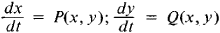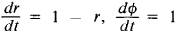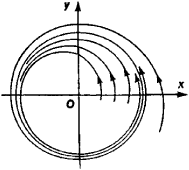# Limit Cycle

(redirected from Limit-cycle)
Also found in: Dictionary, Wikipedia.

## limit cycle

[′lim·ət ‚sīk·əl]
(mathematics)
For a differential equation, a closed trajectory C in the plane (corresponding to a periodic solution of the equation) where every point of C has a neighborhood so that every trajectory through it spirals toward C.

## Limit Cycle

The limit cycle of a system of second-order differential equationsis a closed trajectory in the xy-phase space which has the property that all trajectories starting in a sufficiently narrow annular neighborhood of this trajectory approach it, as t → + ∞ (stable limit cycle) and as t → –∞ (unstable limit cycle), or some approach it as t → + ∞ and the rest as t → —∞ (semistable limit cycle). For example, the system(r and ϕ are polar coordinates), whose general solution is r = 1 — (1 — r0)e-t, ϕ = ϕ0 + t (where r0 ≥ 0), has the stable limit cycle r = 1 (see Figure 1). The concept of limit cycle can be carried over to an nth-order system. From a mechanical viewpoint, a stable limit cycle corresponds to a stable periodic motion of the system. Therefore, finding limit cycles is of great importance in the theory of nonlinear oscillations.Figure 1

### REFERENCES

Pontriagin, L. S. Obyknovennye differentsial’nye urameniia, 3rd ed. Moscow, 1970.
Andronov, A. A., A. A. Vitt, and S. E. Khaikin. Teoriia kolebanii, 2nd ed. Moscow, 1959.
References in periodicals archive ?
2000), a limit-cycle oscillator is a mechanism or system that consistently tends toward specific amplitude and frequency values in its periodic activity.
2000) report observing antiphase activity and mutual arrest among synchronized limit-cycle oscillators once a delay was imposed, a phenomenon they speculate might explain memory lapses.
Adding 8 bits is enough for limit-cycle elimination and reduction of round-off noise to a negligible level.
During the concept-evaluation stage, a single hydraulic actuator with a three-position solenoid-operated on-off valve was modeled, and simulations were performed to determine the relative importance of the various parameters and to study the anticipated limit-cycle behavior.

Site: Follow: Share:
Open / Close